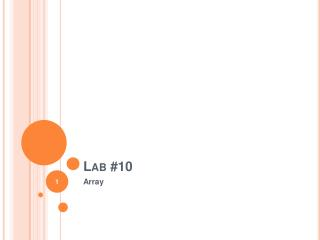DownloadDownload PresentationLab #10

Lab #10

Download PresentationLab #10

- - - - - - - - - - - - - - - - - - - - - - - - - - - E N D - - - - - - - - - - - - - - - - - - - - - - - - - - -
Presentation Transcript

1. Lab #10 Array

2. Passing an Array as a Parameter to a Function #include <iostream>using namespace std;voidprintarray (intarg[], int length) {  for (int n=0; n<length; n++)cout << arg[n] << " ";cout << "\n";}int main (){intfirstarray[] = {5, 10, 15};intsecondarray[] = {2, 4, 6, 8, 10};printarray (firstarray,3);printarray (secondarray,5);return 0;} 5 10 15 2 4 6 8 10

3. What is the output of the following code ? intbilly [] = { 1, 2, 3, 4, 5 }; int a=4; for(inti=0 ; i<5 ; i++) cout<< billy[a-i]<< " " ; cout<<endl; 5 4 3 2 1

4. Write a program that asks the user to type 10 integers of an array. The program must compute and write the number of integers greater or equal to 10. #include<iostream> usingnamespace std; int main() { int limit = 10; int list, count=0; cout<<"Enter 10 integers :"<<endl; for(inti=0; i<limit; i++) { cout<<"Enter Number "<<i+1<<" :"; cin>>list[i]; if (list[i]>=10) count++; } cout<<"Number of interger(s) greater than 10 = "<<count << endl; }

5. Write C++ program that reads 16 numbers from user to initialize two dimensional array then find sum of main diagonal of the array. #include<iostream> usingnamespace std; int main() { intnumArr; int sum = 0, row, col; for (row = 0; row < 4; row++) { for (col = 0; col < 4; col++) { cout << "Enter value for row " << row+1 << " col " << col+1 << ": "; cin >> numArr[row][col]; if (row == col) { sum += numArr[row][col]; } } } cout << "The sum of the values along the main diagonal is: " << sum << endl; }